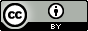Zip in Python

In mathematics, one can have a function $$f: X \times Y\to Z$$ and write $$f(x,y) = z$$, where $$x\in X, y\in Y, z\in Z$$. Some books take care to remark that since $$X \times Y$$ is the domain and $$(x,y)\in X\times Y$$, one should in fact write $$f((x,y))=z$$. In Python, this distinction is accomplished using the star (*) in function calls. So for example if one had a sequence of parameters $$(a_i)_{i=0}^n$$, then one can write $f(\star(a_i)_{i=0}^n) = f(a_{0}, \ldots, a_n)$

Now, the built-in function zip in Python takes the $$i$$th element from each iterable in some sequence, and forms a tuple for each $$i$$, then places all these tuples in one final list. Concretely, suppose we are given some sequence $$x = ((x_{i,j})_{j={0}}^m)_{i={0}}^n$$. Incidentally, this sequence can be visualized as an $$(n+1)\times (m+1)$$ matrix:

$\begin{pmatrix} (x_{0,0}, \ldots, x_{0,m}) \\ \vdots \\ (x_{n,0}, \ldots, x_{n,m}) \end{pmatrix}$

Now let us examine what $$\mathrm{zip}(\star x)$$ means. The star unpacks the first layer of the sequence, leaving us with $\mathrm{zip}((x_{0,0}, \ldots, x_{0,m}), \ldots, (x_{n,0}, \ldots, x_{n,m}))$ Now, $$\mathrm{zip}$$ will first take the $$0$$th element from each argument, forming the sequence $$(x_{0,0},\ldots,x_{n,0})$$; then it will take the elements in position $$1$$ from each argument, forming the sequence $$(x_{0,1}, \ldots, x_{n,1})$$; this process continues until we reach the sequence $$(x_{0,m},\ldots,x_{n,m})$$. Finally, $$\mathrm{zip}$$ will place everything in a sequence of its own, and we obtain a new matrix: $\begin{pmatrix} (x_{0,0},\ldots,x_{n,0}) \\ \vdots \\ (x_{0,m},\ldots,x_{n,m}) \end{pmatrix}$ In other words, everything that was horizontal is now vertical, and the converse holds as well—that is, $$\mathrm{zip}$$ maps each matrix $$x$$ to its transpose $$x^T$$ (or, more specifically, $$\mathrm{zip}$$ maps each matrix $$x = (w_0, \ldots, w_n)^T$$ with row-vectors $$w_0, \ldots, w_n$$ to the matrix $$(w_0^T, \ldots, w_n^T)$$, and then forms row-vectors in the new matrix). If we decide to repeat this operation, we get the original matrix back. In other words, $$x = \mathrm{zip}(\star\mathrm{zip}(\star x))$$.

To give an example of this identity in Python:

>>> x = (1, 2, 3)
>>> y = (4, 5, 6)
>>> lst = [x, y]
>>> lst == zip(*zip(*lst))
True